In mathematics, a quadratic field is a field which is an extension of its prime field of degree two.

In the case when the prime field is finite, so is the quadratic field, and we refer to the article on finite fields. In this article we treat quadratic extensions of the field Q of rational numbers.

In characteristic zero, every quadratic equation is soluble by taking one square root, so a quadratic field is of the form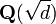$\mathbf{Q}(\sqrt d)$ for a non-zero non-square rational number d. Multiplying by a square integer, we may assume that d is in fact a square-free integer.

## Ring of integers

As above, we take d to be a square-free integer. The maximal order of F is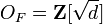$O_F = \mathbf{Z}[\sqrt d] \,$

unless$d \equiv 1 \pmod 4$ in which case$O_F = \mathbf{Z}\left[\frac{1+\sqrt d}{2}\right] .$

### Discriminant

The field discriminant of F is d if$d \equiv 1 \pmod 4$ and otherwise 4d.

### Unit group

If d is negative, then the only units in the ring of integers are roots of unity. If d is positive, the unit group has rank one, with a fundamental unit of infinite order.

## Splitting of primes

The prime 2 is ramified if$d \equiv 2,3 \pmod 4$. If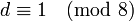$d \equiv 1 \pmod 8$ then 2 splits into two distinct prime ideals, and if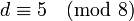$d \equiv 5 \pmod 8$ then 2 is inert.

An odd prime p ramifies iff p divides d. Otherwise, p splits or is inert according as the Legendre symbol$\left(\frac{d}{p}\right)$ is +1 or -1 respectively.

## Galois group

The extension F/Q is generated by the roots of X2d and both roots lie in the field, which is thus a splitting field and so a Galois extension. The Galois group is cyclic of order two, with the non-trivial element being the field automorphism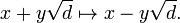$x + y\sqrt d \mapsto x - y\sqrt d .\,$

If F is a complex quadratic field then this automorphism is induced by complex conjugation.